# Algebra 1 : How to find out when an equation has no solution

## Example Questions

### Example Question #1 : How To Find Out When An Equation Has No Solution

Solve the rational equation: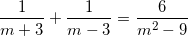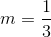no solution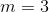or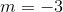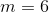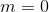no solution

Explanation:

With rational equations we must first note the domain, which is all real numbers exceptand. That is, these are the values ofthat will cause the equation to be undefined. Since the least common denominator of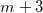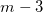, and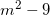is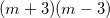, we can mulitply each term by the LCD to cancel out the denominators and reduce the equation to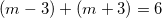. Combining like terms, we end up with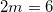. Dividing both sides of the equation by the constant, we obtain an answer of. However, this solution is NOT in the domain. Thus, there is NO SOLUTION becauseis an extraneous answer.

### Example Question #2 : How To Find Out When An Equation Has No Solution

Find the solution set: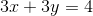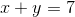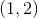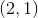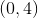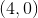Explanation:

Use the substitution method to solve for the solution set.

1)2)Solve equation 2 for y: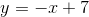Substitute into equation 1: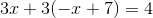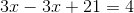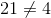If equation 1 was solved for a variable and then substituted into the second equation a similar result would be found. This is because these two equations have No solution. Change both equations into slope-intercept form and graph to visualize. These lines are parallel; they cannot intersect.

*Any method of finding the solution to this system of equations will result in a no solution answer.

### Example Question #3 : How To Find Out When An Equation Has No Solution

How many solutions does the equation below have?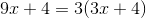One

Three

No solutions

Infinite

Two

No solutions

Explanation:

When finding how many solutions an equation has you need to look at the constants and coefficients.

The coefficients are the numbers alongside the variables.

The constants are the numbers alone with no variables.

If the coefficients are the same on both sides then the sides will not equal, therefore no solutions will occur.Use distributive property on the right side first.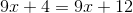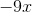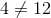No solutions

### Example Question #4 : How To Find Out When An Equation Has No Solution

Solve: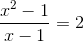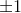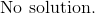Explanation:

First factorize the numerator.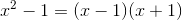Rewrite the equation.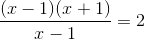The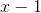terms can be eliminated.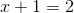Subtract one on both sides.However, let's substitute this answer back to the original equation to check whether if we will getas an answer.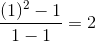Simplify the left side.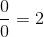The left side does not satisfy the equation because the fraction cannot be divided by zero.

Therefore,is not valid.

The answer is:### Example Question #1 : How To Find Out When An Equation Has No Solution

Solve for: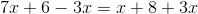No solutionNo solution

Explanation:

Combine like terms on each side of the equation: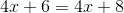Next, subtractfrom both sides.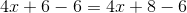Then subtract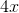from both sides.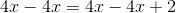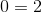This is nonsensical; therefore, there is no solution to the equation.

### Example Question #6 : How To Find Out When An Equation Has No Solution

Solve the equation: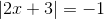No solutionNo solution

Explanation:

Notice that the end value is a negative.  Any negative or positive value that is inside an absolute value sign must result to a positive value.

If we split the equation to its positive and negative solutions, we have: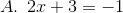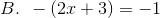Solve the first equation.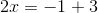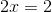The answer to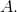is:Solve the second equation.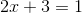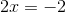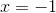The answer to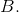is:If we substitute these two solutions back to the original equation, the results are positive answers and can never be equal to negative one.